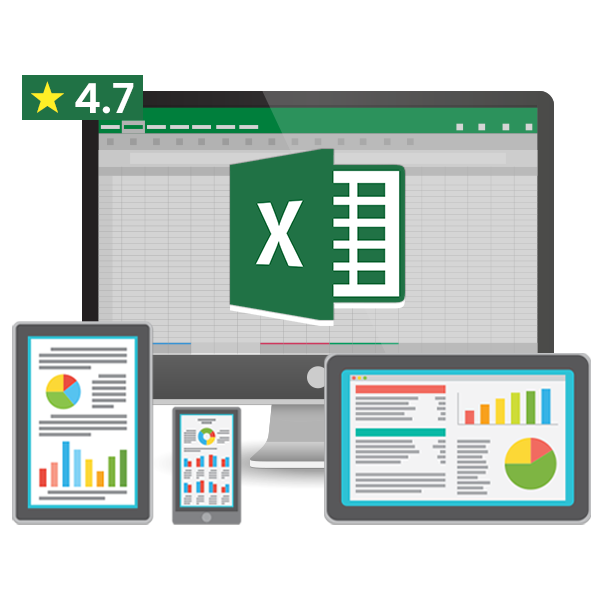# Average function in excel## How to use the Average function in excel

This tutorial will give you a detailed description regarding the Average in Excel. The average formula is the most used function after Autosum. This tutorial will give you knowledge regarding:

• What is average in Excel?
• What are the uses of average in Excel?
• What is the formula to calculate average?
• What is the purpose of using average in excel?
• When and where can average in excel be used?
• Requirements and non-requirement for calculate average?
• What are the ways or methods to calculate average?

Let’s take a detailed look into this function.Full Excel Course in Hindi

• Get 2-Year Access with unlimited views of 220+ videos
• Get Course Completion Certificate & Excel Mastery eBooks

What is the average in MS Excel?

This function is used to calculate the arithmetic mean of the numbers, arguments, cell references, range, etc. The average is the inbuilt function in Microsoft Excel. It is a statistical function in Excel. It is entered in the formula bar of Excel. Whenever we hear the word average the first thing that comes in mind is the arithmetic mean of the numbers. The average formula can handle up to 255 arguments.

What is the purpose of the average?

The purpose of this function is to get the average of group of numbers. Another purpose of using average function is to get the average numeric value for our representation. Average is a worksheet function which is used to carry out functions in our worksheet. This function makes it easier for mathematical calculation. This function is the most required function while working in the worksheet.

What is the formula to calculate the average?

There is a particular syntax that is to be carried out while performing or calculating average for the given numbers. Like to calculate average we will add all the numbers and then divide the total by the count numbers. For example, there are five numbers 7,8,9,10,11 we need to count the average for this function. We will do so by adding all the above numbers (7+8+9+10+11) will be 45. The total numbers are 5. So the arithmetic mean will be 45 divide by 5 which will be 9.

The syntax of Average Function in Excel:

=Average(Number1, [Number 2]…)

Where number 1 and number 2 are the numeric values you want to find average of. The number 1 is required to be filled and rest all is optional. You can add 255 numbers in a single formula.
Suppose we want to count the above example in Excel then we must syntax it as =Average (A1, A2, A3, A4, A5) the answer will be the same 9.

You can also insert a range of cells. Like for the above example, you can count average by using the following syntax
=average(A1:A5)

Points to Remember:

• The arguments should only be of numbers.
• The number 0 will be included while calculating the average.
• The blank cell will not be taken into consideration while calculating the average.
• The cell should not contain text or any logical values, they will be ignored.
• If we want to include the text representation of numbers then we must use AVERAGEA function.
• If we want to calculate the average of the numbers that meet certain criteria then we must use the AVERAGEIF function.
• The = equal to sign is a must while carrying out average, otherwise, other function will be enabled.

Where do you find the average function in MS Excel?

You will find this function in the AutoSum drop down menu. AutoSum drop down menu is at the home ribbon editing group with the AutoSum name and sign ∑.

Another place to find Average formula is when you press fx function above the spreadsheet and below the ribbon. As you press this function an Insert function dialog box will open. There you can select the function average.

The third place you find Average is in the formula ribbon. There you find Autosum and as u click on the drop down it will show Average. You can also go into the function library group where you find more function. More function will show statistical and as you press the right arrow key it will show the average functions.

However the shortcut to insert average formula through keyboard is Shift + F3. This shortcut key will open the insert function dialog box and you select average.

Conclusion:

The above tutorial gave you the basic idea about the average formula use in Excel. I hope you might have understood about the Average Excel function. Just remember that by term average is Excel is the arithmetic mean and not the central tendency mean or the standard deviation mean. It is just a simple mean or average to calculate the middle or the center part of the worksheet.